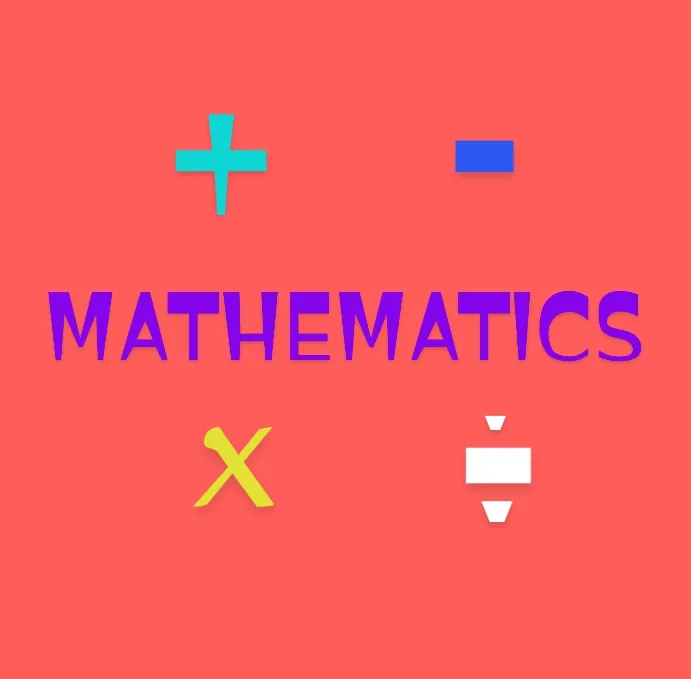Learning edition 2: MATHEMATICSPlan: creating an educational content every day , each day with different subject and different topic

Purpose: Promoting education in this community for members of this community everyday for this community growth.

You can ask any question under the topic discussed in the comment session

Subject available are ENGLISH LANGUAGE, BIOLOGY , MATHEMATICS, CHEMISTRY ,PHYSICS , ECONOMICS , AGRICULTURE,SPORT and CRYPTOCURRENCY

Today's #topic

SURDS

Numbers like 2,2.4,0.3.0.6,√64 can be expressed as exact fractions or ratios:5/1,1/3 ,3/5,8 . These are rational numbers.
But Numbers that cannot be written in ratio form are known as irrational numbers.
For example **π= 3.1415........,
Other example of irrational numbers are √5= 2.236067977..., and √7 = 2.645751....,
Numbers that are irrational are called SURD.
Two or more surd are called surds if the numbers under square root sign and the same, for example **√5 , 3√3 , √3/2 are called surds.

Simplification of surds

To simplify a surd, where possible, Express the number under the square root sign as a product of two factors, one which is a perfect square.
Then simplify the surd by taking the square root of the perfect square.
EXAMPLE 1
To simplify √20
**step **
Express the number under the square root sign as a product of two factors as in
√20= √4x√5
then simplify
√20= 2x√5
=2√5

EXAMPLE 2
To simplify 3√50
step
Express the number under the square root sign as a product of two factors as in
3√50= 3x√25x√2
Notice that this is more complex than the first one
You should not be confused by the 3. It is just multiplied by the √50.
So you can just solve that of √50 and multiplied by 3
3√50= 3x5x√2
=15√2

YOU CAN ASK ANY QUESTION IN THE COMMENT SECTION
SOLVE THIS QUESTION IN THE COMMENT SESSION
SIMPLIFY THE FOLLOWING
1, √162
2, √1000
3, 2√500